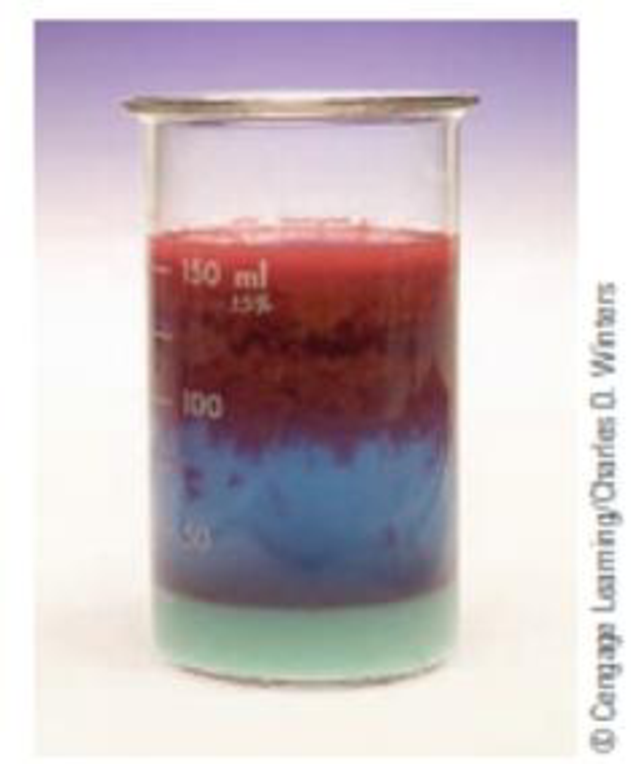Chapter 13, Problem 57GQ

Chapter
Section
Textbook Problem

Dimethylglyoxime [DMG, (CH3CNOH)2] is used as a reagent to precipitate nickel ion. Assume that 53.0 g of DMC has been dissolved in 525 g of ethanol (C2H5OH).sThe red, insoluble compound formed between nickel(II)ion and dimethylglyoxime (DMG) is precipitated when DMG is added to a bask solution of Ni2+ (aq). (a) What is the mole fraction of DMG? (b) What is the molality of the solution? (c) What is the vapor pressure of the ethanol over the solution at ethanol’s normal boiling point of 78.4 °C? (d) What is the boiling point of the solution? (DMG does not produce ions in solution.) (Kbp for ethanol = +1.22 °C/m)

(a)

Interpretation Introduction

Interpretation: Mole fraction of DMG has to be calculated.

Concept introduction:

Raoult’s law: In a solution, vapor pressure of solvent is proportional to its mole fraction.

Psolvent=XsolventP0solvent

where,

P0solvent is the vapor pressure of pure solvent.

Mole fraction: Amount of that component divided by the total amount of all of the components of the mixture ( nA +  nB +  n...).

Mole fraction of A (χA)= nA nA +  nB +  n...

Explanation

Given,

Massofethanol=53.0gMassofDMG=525gMolarmassofethanol=46.07g/molMolarmassofDMG=116.12g/mol

The number of moles of any substance can be determined using the equation

Numberofmole=GivenmassofthesubstanceMolarmass

Number of moles of dimethylglyoxime is,

nDMG=53.0g116.12g/mol=0

(b)

Interpretation Introduction

Interpretation: Molality of the solution has to be determined.

Concept introduction:

Raoult’s law: In a solution, vapor pressure of solvent is proportional to its mole fraction.

Psolvent=XsolventP0solvent

where,

P0solvent is the vapor pressure of pure solvent.

Mole fraction: Amount of that component divided by the total amount of all of the components of the mixture ( nA +  nB +  n...).

Mole fraction of A (χA)= nA nA +  nB +  n...

(c)

Interpretation Introduction

Interpretation: Mole fraction of DMG and molality of the solution are to be determined. Vapour pressure of ethanol over the solution and Boiling point of the solution are to be calculated.

Concept introduction:

Raoult’s law: In a solution, vapor pressure of solvent is proportional to its mole fraction.

Psolvent=XsolventP0solvent

where,

P0solvent is the vapor pressure of pure solvent.

Mole fraction: Amount of that component divided by the total amount of all of the components of the mixture ( nA +  nB +  n...).

Mole fraction of A (χA)= nA nA +  nB +  n...

(d)

Interpretation Introduction

Interpretation: Mole fraction of DMG and molality of the solution are to be determined. Vapour pressure of ethanol over the solution and Boiling point of the solution are to be calculated.

Concept introduction:

Raoult’s law: In a solution, vapor pressure of solvent is proportional to its mole fraction.

Psolvent=XsolventP0solvent

where,

P0solvent is the vapor pressure of pure solvent.

Mole fraction: Amount of that component divided by the total amount of all of the components of the mixture ( nA +  nB +  n...).

Mole fraction of A (χA)= nA nA +  nB +  n...

Still sussing out bartleby?

Check out a sample textbook solution.

See a sample solution

The Solution to Your Study Problems

Bartleby provides explanations to thousands of textbook problems written by our experts, many with advanced degrees!

Get Started

Find more solutions based on key concepts